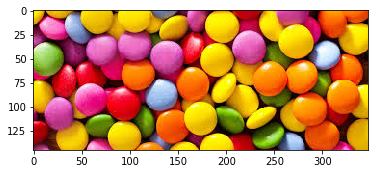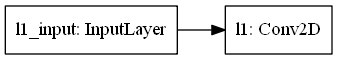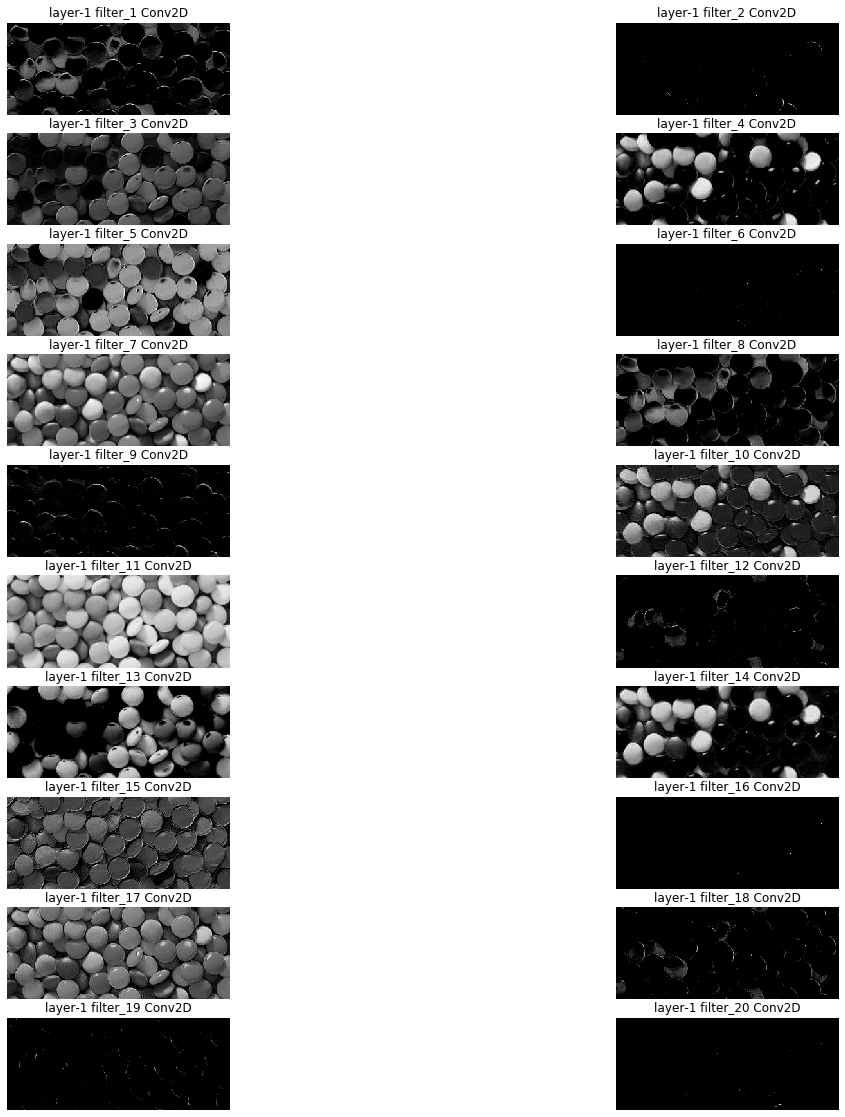In :
``````import cv2 as cv
import matplotlib.pyplot as plt
import numpy as np
import tensorflow as tf``````

### Read in image

In :
``````im = cv.imread("Candy.jfif")
im = im[:,:,::-1]
plt.imshow(im)
``````
Out:
``<matplotlib.image.AxesImage at 0x26730721408>``### Load keras's essential libraries

for number of filters??: https://stackoverflow.com/questions/36243536/what-is-the-number-of-filter-in-cnn

In :
``````import keras
from keras.models import Sequential
from keras.layers import Conv2D,MaxPooling2D,Flatten
from keras.layers import Dense
from keras import backend as k
from keras.utils import plot_model``````
```Using TensorFlow backend. ```

### This below function is must to reproduce same results in each run

###### !!!!!!
In :
``````def reproduceResult():
# Seed value (can actually be different for each attribution step)
seed_value= 0

# 1. Set `PYTHONHASHSEED` environment variable at a fixed value
import os
os.environ['PYTHONHASHSEED']=str(seed_value)

# 2. Set `python` built-in pseudo-random generator at a fixed value
import random
random.seed(seed_value)

# 3. Set `numpy` pseudo-random generator at a fixed value
import numpy as np
np.random.seed(seed_value)

# 4. Set `tensorflow` pseudo-random generator at a fixed value
import tensorflow as tf
tf.compat.v1.set_random_seed(seed_value)
``````

### Generate sequential model and add layers in it

There is no correct answer as to what the best number of filters is. This strongly depends on the type and complexity of your (image) data. A suitable number of features is learnd from experience after working with similar types of datasets repeatedly over time. In general, the more features you want to capture (and are potentially available) in an image the higher the number of filters required in a CNN.

##### lets use filter size as 20 and see what happens in each filter
In :
``````reproduceResult()
X = im[:,:,::-1]
model = Sequential(name="s1")
model.add(Conv2D(20, kernel_size=(2,2),strides=(1, 1),activation='relu',input_shape=X.shape,name="l1"))

plot_model(model,rankdir="LR")

im_batch = np.expand_dims(im[:,:,::-1],axis=0)
# print(im_batch.shape)
im_conv = model.predict(im_batch,verbose=0)

op_conv = np.squeeze(im_conv) # to make it 2d array
print(op_conv.shape)
``````
```(144, 347, 20) ```

### Print summary of model

In :
``X.shape``
Out:
``(145, 348, 3)``
In :
``model.summary()``
```Model: "s1" _________________________________________________________________ Layer (type) Output Shape Param # ================================================================= l1 (Conv2D) (None, 144, 347, 20) 260 ================================================================= Total params: 260 Trainable params: 260 Non-trainable params: 0 _________________________________________________________________ ```

### Visualize the model

In :
``plot_model(model,rankdir="LR")``
Out:### Display final output of colvolved image

In :
``op_conv.shape``
Out:
``(144, 347, 20)``
In :
``model.layers.filters``
Out:
``20``

### Visualize each filter output

In :
``````plt.figure(figsize=(20,20))
plt.tight_layout()
for i in range(1,model.layers.filters+1):
plt.subplot(10,2,i)
plt.imshow(op_conv[:,:,i-1],cmap="gray")
plt.title("layer-1 "+"filter_"+str(i)+" "+str(model.get_layer("l1")).split(".")[-1].split(" "))
plt.axis("off")
plt.show()
``````In :
``model.get_weights().shape``
Out:
``(2, 2, 3, 20)``